# NCERT Solutions for Class 9th: Ch 7 Triangles Maths

#### NCERT Solutions for Class 9th: Ch 7 Triangles Maths

Page No: 118

Exercise 7.1

1. In quadrilateral ACBD, AC = AD and AB bisects ∠A (see Fig. 7.16). Show that ΔABC ≅ ΔABD. What can you say about BC and BD?Given,
AC = AD and AB bisects ∠A
To prove,
ΔABC ≅ ΔABD
Proof,
In ΔABC and ΔABD,
AB = AB (Common)
∠CAB = ∠DAB (AB is bisector)
Therefore, ΔABC ≅ ΔABD by SAS congruence condition.
BC and BD are of equal length.

Page No: 119

2. ABCD is a quadrilateral in which AD = BC and ∠DAB = ∠CBA (see Fig. 7.17). Prove that
(i) ΔABD ≅ ΔBAC
(ii) BD = AC
(iii) ∠ABD = ∠BAC.Given,
AD = BC and ∠DAB = ∠CBA

(i) In ΔABD and ΔBAC,
AB = BA (Common)
∠DAB = ∠CBA (Given)
Therefore, ΔABD ≅ ΔBAC by SAS congruence condition.
(ii) Since, ΔABD ≅ ΔBAC
Therefore BD = AC by CPCT
(iii) Since, ΔABD ≅ ΔBAC
Therefore ∠ABD = ∠BAC by CPCT

3. AD and BC are equal perpendiculars to a line segment AB (see Fig. 7.18). Show that CD bisects AB.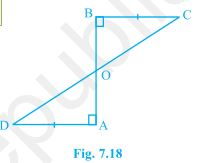Given,
AD and BC are equal perpendiculars to AB.
To prove,
CD bisects AB
Proof,
In ΔAOD and ΔBOC,
∠A = ∠B (Perpendicular)
∠AOD = ∠BOC (Vertically opposite angles)
Therefore, ΔAOD ≅ ΔBOC by AAS congruence condition.
Now,
AO = OB (CPCT). CD bisects AB.

4. l and m are two parallel lines intersected by another pair of parallel lines p and q (see Fig. 7.19). Show that ΔABC ≅ ΔCDA.Given,
l || m and p || q
To prove,
ΔABC ≅ ΔCDA
Proof,
In ΔABC and ΔCDA,
∠BCA = ∠DAC (Alternate interior angles)
AC = CA (Common)
∠BAC = ∠DCA (Alternate interior angles)
Therefore, ΔABC ≅ ΔCDA by ASA congruence condition.

5. Line l is the bisector of an angle ∠A and B is any point on l. BP and BQ are perpendiculars from B to the arms of ∠A (see Fig. 7.20). Show that:
(i) ΔAPB ≅ ΔAQB
(ii) BP = BQ or B is equidistant from the arms of ∠A.Given,
l is the bisector of an angle ∠A.
BP and BQ are perpendiculars.

(i) In ΔAPB and ΔAQB,
∠P = ∠Q (Right angles)
∠BAP = ∠BAQ (l is bisector)
AB = AB (Common)
Therefore, ΔAPB ≅ ΔAQB by AAS congruence condition.
(ii) BP = BQ by CPCT. Therefore, B is equidistant from the arms of ∠A.

Page No: 120

6. In Fig. 7.21, AC = AE, AB = AD and ∠BAD = ∠EAC. Show that BC = DE.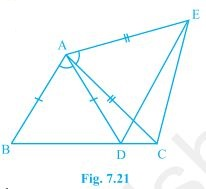Given,
To show,
BC = DE
Proof,
∠BAD + ∠DAC = ∠EAC + ∠DAC
AC = AE (Given)
Therefore, ΔABC ≅ ΔADE by SAS congruence condition.
BC = DE by CPCT.

7. AB is a line segment and P is its mid-point. D and E are points on the same side of AB such that ∠BAD = ∠ABE and ∠EPA = ∠DPB (see Fig. 7.22). Show that
(i) ΔDAP ≅ ΔEBPGiven,
P is mid-point of AB.
∠BAD = ∠ABE and ∠EPA = ∠DPB

(i) ∠EPA = ∠DPB (Adding ∠DPE both sides)
∠EPA + ∠DPE = ∠DPB + ∠DPE
⇒ ∠DPA = ∠EPB
In ΔDAP ≅ ΔEBP,
∠DPA = ∠EPB
AP = BP (P is mid-point of AB)
Therefore, ΔDAP ≅ ΔEBP by ASA congruence condition.
(ii) AD = BE by CPCT.

8. In right triangle ABC, right angled at C, M is the mid-point of hypotenuse AB. C is joined to M and produced to a point D such that DM = CM. Point D is joined to point B (see Fig. 7.23). Show that:
(i) ΔAMC ≅ ΔBMD
(ii) ∠DBC is a right angle.
(iii) ΔDBC ≅ ΔACB
(iv) CM = 1/2 ABGiven,
∠C = 90°, M is the mid-point of AB and DM = CM

(i) In ΔAMC and ΔBMD,
AM = BM (M is the mid-point)
∠CMA = ∠DMB (Vertically opposite angles)
CM = DM (Given)
Therefore, ΔAMC ≅ ΔBMD by SAS congruence condition.

(ii) ∠ACM = ∠BDM (by CPCT)
Therefore, AC || BD as alternate interior angles are equal.
Now,
∠ACB ∠DBC = 180° (co-interiors angles)
⇒ 90° + ∠B = 180°
⇒ ∠DBC = 90°

(iii) In ΔDBC and ΔACB,
BC = CB (Common)
∠ACB = ∠DBC (Right angles)
DB = AC (byy CPCT, already proved)
Therefore, ΔDBC ≅ ΔACB by SAS congruence condition.

(iv)  DC = AB (ΔDBC ≅ ΔACB)
⇒ DM = CM = AM = BM (M is mid-point)
⇒ DM + CM = AM + BM
⇒ CM + CM = AB
⇒ CM = 1/2AB

Page No: 123

Exercise 7.2

1. In an isosceles triangle ABC, with AB = AC, the bisectors of ∠B and ∠C intersect each other at O. Join A to O. Show that :
(i) OB = OC                     (ii) AO bisects ∠AGiven,
AB = AC, the bisectors of ∠B and ∠C intersect each other at O

(i) Since ABC is an isosceles with AB = AC,
∴ ∠B = ∠C
⇒ 1/2∠B = 1/2∠C
⇒ ∠OBC = ∠OCB (Angle bisectors.)
⇒ OB = OC (Side opposite to the equal angles are equal.)

(ii) In ΔAOB and ΔAOC,
AB = AC (Given)
AO = AO (Common)
OB = OC (Proved above)
Therefore, ΔAOB ≅ ΔAOC by SSS congruence condition.
∠BAO = ∠CAO (by CPCT)
Thus, AO bisects ∠A.

2. In ΔABC, AD is the perpendicular bisector of BC (see Fig. 7.30). Show that ΔABC is an isosceles triangle in which AB = AC.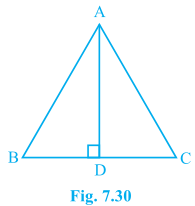Given,
AD is the perpendicular bisector of BC
To show,
AB = AC
Proof,
BD = CD (AD is the perpendicular bisector)
AB = AC (by CPCT)

Page No: 124

3. ABC is an isosceles triangle in which altitudes BE and CF are drawn to equal sides AC and AB respectively (see Fig. 7.31). Show that these altitudes are equal.Given,
BE and CF are altitudes.
AC = AB
To show,
BE = CF
Proof,
In ΔAEB and ΔAFC,
∠A = ∠A (Common)
∠AEB = ∠AFC (Right angles)
AB = AC (Given)
Therefore, ΔAEB ≅ ΔAFC by AAS congruence condition.
Thus, BE = CF by CPCT.

4. ABC is a triangle in which altitudes BE and CF to sides AC and AB are equal (see Fig. 7.32). Show that
(i) ΔABE ≅ ΔACF
(ii) AB = AC, i.e., ABC is an isosceles triangle.Given,
BE = CF

(i) In ΔABE and ΔACF,
∠A = ∠A (Common)
∠AEB = ∠AFC (Right angles)
BE = CF (Given)
Therefore, ΔABE ≅ ΔACF by AAS congruence condition.

(ii) Thus, AB = AC by CPCT and therefore ABC is an isosceles triangle.

5. ABC and DBC are two isosceles triangles on the same base BC (see Fig. 7.33). Show that ∠ABD = ∠ACD.Given,
ABC and DBC are two isosceles triangles.
To show,
∠ABD = ∠ACD
Proof,
In ΔABD and ΔACD,
AB = AC (ABC is an isosceles triangle.)
BD = CD (BCD is an isosceles triangle.)
Therefore, ΔABD ≅ ΔACD by SSS congruence condition. Thus, ∠ABD = ∠ACD by CPCT.

6. ΔABC is an isosceles triangle in which AB = AC. Side BA is produced to D such that AD = AB (see Fig. 7.34). Show that ∠BCD is a right angle.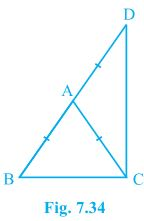Given,
AB = AC and AD = AB
To show,
∠BCD is a right angle.
Proof,
In ΔABC,
AB = AC (Given)
⇒ ∠ACB = ∠ABC (Angles opposite to the equal sides are equal.)
In ΔACD,
⇒ ∠ADC = ∠ACD (Angles opposite to the equal sides are equal.)
Now,
In ΔABC,
∠CAB + ∠ACB ∠ABC = 180°
⇒ ∠CAB + 2∠ACB = 180°
⇒ ∠CAB = 180° - 2∠ACB --- (i)
∠CAD = 180° - 2∠ACD --- (ii)
also,
∠CAB + ∠CAD = 180° (BD is a straight line.)
∠CAB + ∠CAD = 180° - 2∠ACB + 180° - 2∠ACD
⇒ 180° = 360° - 2∠ACB - 2∠ACD
⇒ 2(∠ACB + ∠ACD) = 180°
⇒ ∠BCD = 90°

7. ABC is a right angled triangle in which ∠A = 90° and AB = AC. Find ∠B and ∠C.

Given,
∠A = 90° and AB = AC
A/q,
AB = AC
⇒ ∠B = ∠C (Angles opposite to the equal sides are equal.)
Now,
∠A + ∠B + ∠C = 180° (Sum of the interior angles of the triangle.)
⇒ 90° + 2∠B = 180°
⇒ 2∠B = 90°
⇒ ∠B = 45°
Thus, ∠B = ∠C = 45°

8. Show that the angles of an equilateral triangle are 60° each.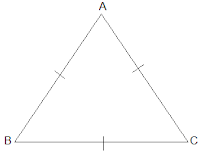Let ABC be an equilateral triangle.
BC = AC = AB (Length of all sides is same)
⇒ ∠A = ∠B = ∠C (Sides opposite to the equal angles are equal.)
Also,
∠A + ∠B + ∠C = 180°
⇒ 3∠A = 180°
⇒ ∠A = 60°
Therefore, ∠A = ∠B = ∠C = 60°
Thus, the angles of an equilateral triangle are 60° each.

Page No: 128

Exercise 7.3

1. ΔABC and ΔDBC are two isosceles triangles on the same base BC and vertices A and D are on the same side of BC (see Fig. 7.39). If AD is extended to intersect BC at P, show that
(i) ΔABD ≅ ΔACD
(ii) ΔABP ≅ ΔACP
(iii) AP bisects ∠A as well as ∠D.
(iv) AP is the perpendicular bisector of BC.Given,
ΔABC and ΔDBC are two isosceles triangles.

(i) In ΔABD and ΔACD,
AB = AC (ΔABC is isosceles)
BD = CD (ΔDBC is isosceles)
Therefore, ΔABD ≅ ΔACD by SSS congruence condition.

(ii) In ΔABP and ΔACP,
AP = AP (Common)
∠PAB = ∠PAC (ΔABD ≅ ΔACD so by CPCT)
AB = AC (ΔABC is isosceles)
Therefore, ΔABP ≅ ΔACP by SAS congruence condition.

(iii) ∠PAB = ∠PAC by CPCT as ΔABD ≅ ΔACD.
AP bisects ∠A. --- (i)
also,
In ΔBPD and ΔCPD,
PD = PD (Common)
BD = CD (ΔDBC is isosceles.)
BP = CP (ΔABP ≅ ΔACP so by CPCT.)
Therefore, ΔBPD ≅ ΔCPD by SSS congruence condition.
Thus, ∠BDP = ∠CDP by CPCT. --- (ii)
By (i) and (ii) we can say that AP bisects ∠A as well as ∠D.

(iv) ∠BPD = ∠CPD (by CPCT as ΔBPD ≅ ΔCPD)
and BP = CP --- (i)
also,
∠BPD + ∠CPD = 180° (BC is a straight line.)
⇒ 2∠BPD = 180°
⇒ ∠BPD = 90° ---(ii)
From (i) and (ii),
AP is the perpendicular bisector of BC.

2. AD is an altitude of an isosceles triangle ABC in which AB = AC. Show thatGiven,
AD is an altitude and AB = AC

(i) In ΔABD and ΔACD,
AB = AC (Given)
Therefore, ΔABD ≅ ΔACD by RHS congruence condition.
Now,
BD = CD (by CPCT)

3. Two sides AB and BC and median AM of one triangle ABC are respectively equal to sides PQ and QR and median PN of ΔPQR (see Fig. 7.40). Show that:
(i) ΔABM ≅ ΔPQN
(ii) ΔABC ≅ ΔPQR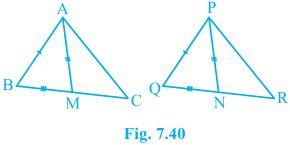Given,
AB = PQ, BC = QR and AM = PN

(i) 1/2 BC = BM and 1/2QR = QN (AM and PN are medians)
also,
BC = QR
⇒ 1/2 BC = 1/2QR
⇒ BM = QN
In ΔABM and ΔPQN,
AM = PN (Given)
AB = PQ (Given)
BM = QN (Proved above)
Therefore, ΔABM ≅ ΔPQN by SSS congruence condition.

(ii) In ΔABC and ΔPQR,
AB = PQ (Given)
∠ABC = ∠PQR (by CPCT)
BC = QR (Given)

Therefore, ΔABC ≅ ΔPQR by SAS congruence condition.

4. BE and CF are two equal altitudes of a triangle ABC. Using RHS congruence rule, prove that the triangle ABC is isosceles.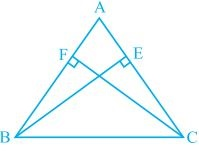Given,
BE and CF are two equal altitudes.
In ΔBEC and ΔCFB,
∠BEC = ∠CFB = 90° (Altitudes)
BC = CB (Common)
BE = CF (Common)
Therefore, ΔBEC ≅ ΔCFB by RHS congruence condition.
Now,
∠C = ∠B (by CPCT)
Thus, AB = AC as sides opposite to the equal angles are equal.

5. ABC is an isosceles triangle with AB = AC. Draw AP ⊥ BC to show that ∠B = ∠C.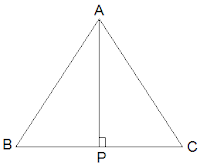Given,
AB = AC
In ΔABP and ΔACP,
∠APB = ∠APC = 90° (AP is altitude)
AB = AC (Given)
AP = AP (Common)
Therefore, ΔABP ≅ ΔACP by RHS congruence condition.
Thus, ∠B = ∠C (by CPCT)

Page No: 132

Exercise 7.4

1. Show that in a right angled triangle, the hypotenuse is the longest side.ABC is a triangle right angled at B.
Now,
∠A ∠B ∠C = 180°
⇒ ∠A + ∠C = 90° and ∠B is 90°.
Since, B is the largest angle of the triangle, the side opposite to it must be the largest.
So, BC is the hypotenuse which is the largest side of the right angled triangle ABC.

2. In Fig. 7.48, sides AB and AC of ΔABC are extended to points P and Q respectively. Also, ∠PBC < ∠QCB. Show that AC > AB.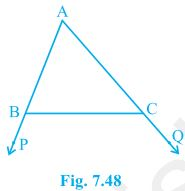Given,
∠PBC < ∠QCB
Now,
∠ABC + ∠PBC = 180°
⇒ ∠ABC = 180° - ∠PBC
also,
∠ACB + ∠QCB = 180°
⇒ ∠ACB = 180° - ∠QCB
Since,
∠PBC < ∠QCB therefore, ∠ABC > ∠ACB
Thus, AC > AB as sides opposite to the larger angle is larger.

3. In Fig. 7.49, ∠B < ∠A and ∠C < ∠D. Show that AD < BC.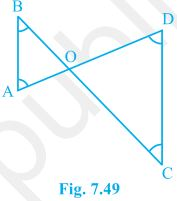Given,
∠B < ∠A and ∠C < ∠D
Now,
AO <  BO --- (i) (Side opposite to the smaller angle is smaller)
OD < OC ---(ii) (Side opposite to the smaller angle is smaller)
AO + OD < BO + OC

4. AB and CD are respectively the smallest and longest sides of a quadrilateral ABCD (see Fig. 7.50).
Show that ∠A > ∠C and ∠B > ∠D.In ΔABD,
∴ ∠ADB < ∠ABD --- (i) (Angle opposite to longer side is larger.)
Now,
In ΔBCD,
BC < DC < BD
∴ ∠BDC < ∠CBD --- (ii)
Adding (i) and (ii) we get,
∠ADB + ∠BDC < ∠ABD + ∠CBD
⇒ ∠B > ∠D
Similarly,
In ΔABC,
∠ACB < ∠BAC --- (iii) (Angle opposite to longer side is larger.)
Now,
∠DCA < ∠DAC --- (iv)
Adding (iii) and (iv) we get,
∠ACB + ∠DCA < ∠BAC + ∠DAC
⇒ ∠A > ∠C

5. In Fig 7.51, PR > PQ and PS bisects ∠QPR. Prove that ∠PSR > ∠PSQ.Given,
PR > PQ and PS bisects ∠QPR
To prove,
∠PSR > ∠PSQ
Proof,
∠PQR > ∠PRQ --- (i) (PR > PQ as angle opposite to larger side is larger.)
∠QPS = ∠RPS --- (ii) (PS bisects ∠QPR)
∠PSR = ∠PQR ∠QPS --- (iii) (exterior angle of a triangle equals to the sum of opposite interior angles)
∠PSQ = ∠PRQ + ∠RPS --- (iv) (exterior angle of a triangle equals to the sum of opposite interior angles)
∠PQR + ∠QPS > ∠PRQ + ∠RPS
⇒ ∠PSR > ∠PSQ [from (i), (ii), (iii) and (iv)]

Page No: 133

6. Show that of all line segments drawn from a given point not on it, the perpendicular line segment is the shortest.